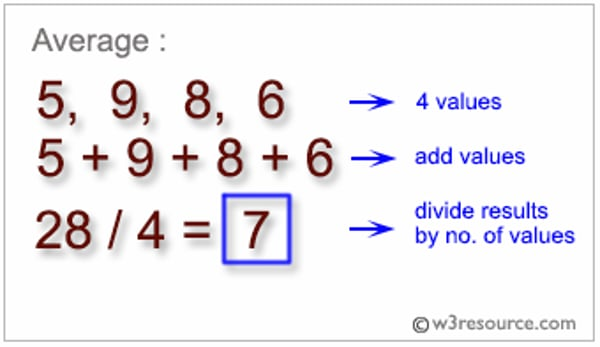Related Tags

python

# How to calculate the average of a list of numbers in Python

Sometimes, it is necessary to find the average of a given list of numbers.Calculating average

In Python, we can calculate the average of a list using the sum and length of the list.

1.sum()

2.len()

3.numpy.average

import numpy as np
# list l1
l1 = [5, 9, 8, 6]

# length of the list
length = len(l1)

# sum of values of the list
sum_list = sum(l1)

# Average
avg = sum_list / length

print("Average =", avg)

## using numpy
data = np.array(l1)

print("avg using numpy = ", np.average(data))

RELATED TAGS

python

CONTRIBUTOR